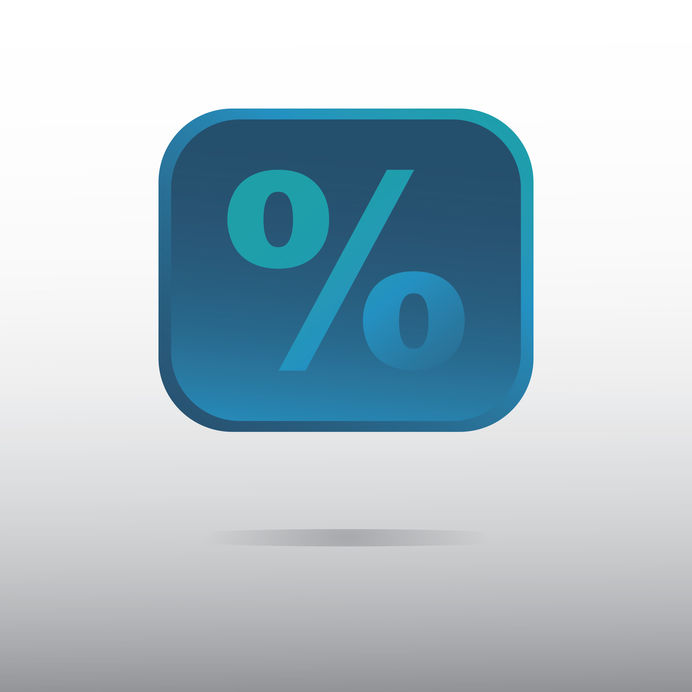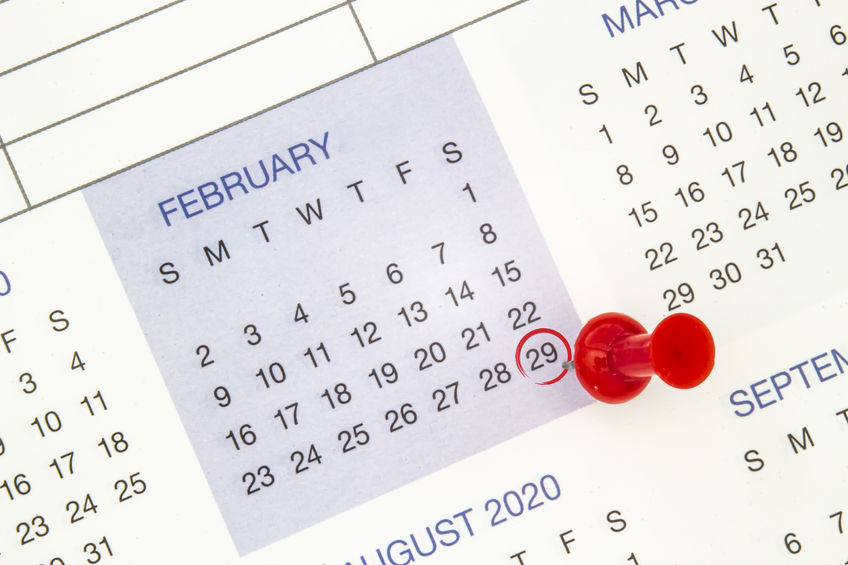# The Blog of Mathedu Academy

### A content for the curious of the mathematics in the real world… and beyond, including video tutorials.# Add a percentage is a Multiplication

Add a percentage, say 5% is not making an addition. It is a multiplication by (1+5/100). You may also multiply by 5, divide by 100, and then add the result to the original amount.# Manipulate percentages like a Pro

We learned in the previous post to add a percentage with a multiplication, let’s now learn the different manipulations of percentage.# The Roman Numerals, an alternative numeration system.

The Roman Numerals System is an alternative numeric system, that was invented by the Romans and is still used in some cases, such as… the Star Wars episodes numbering!